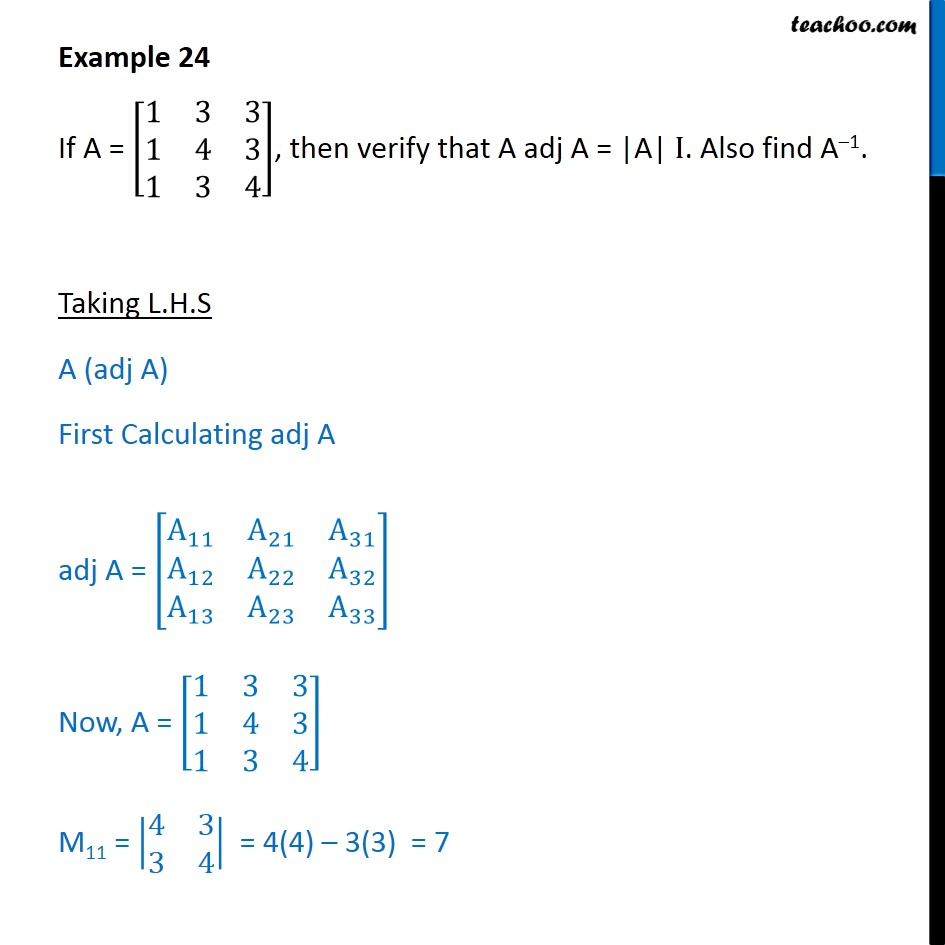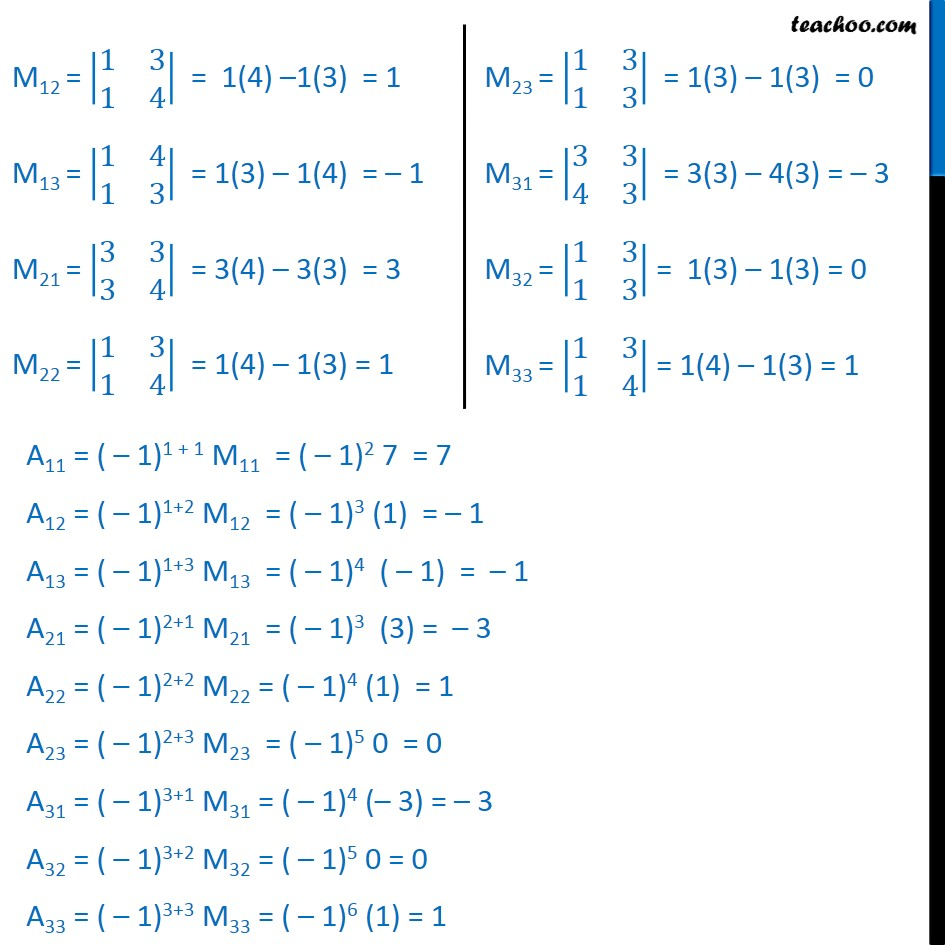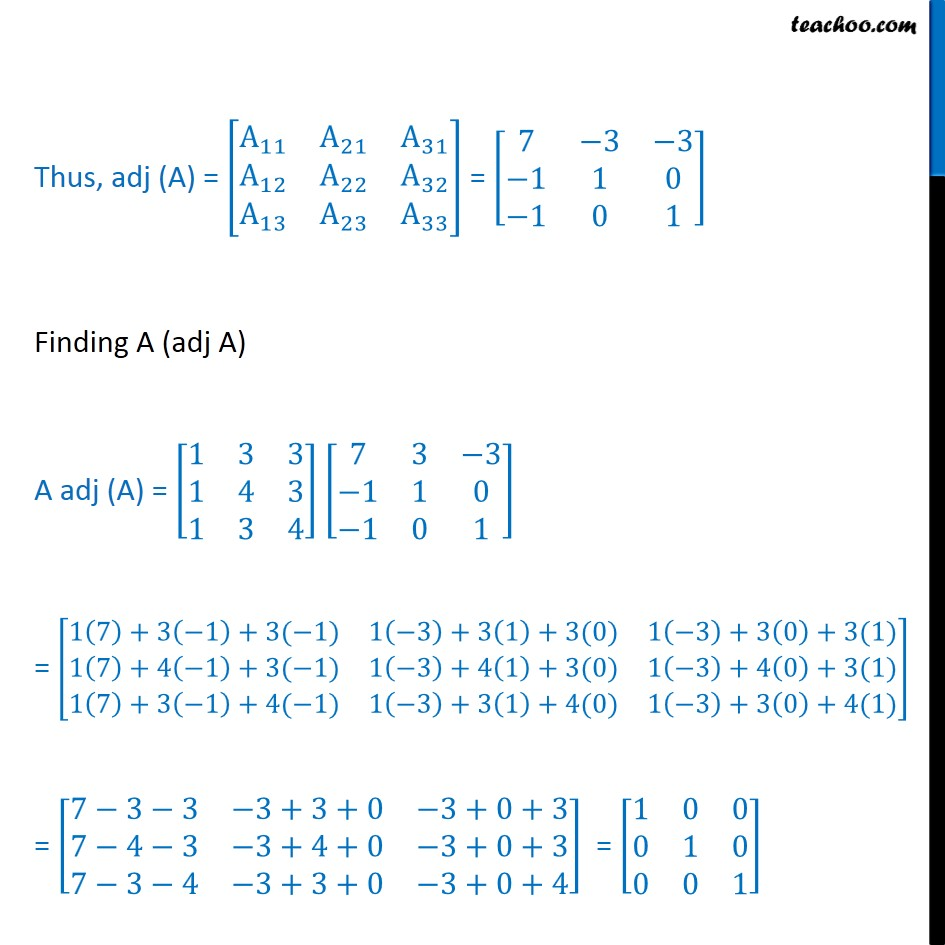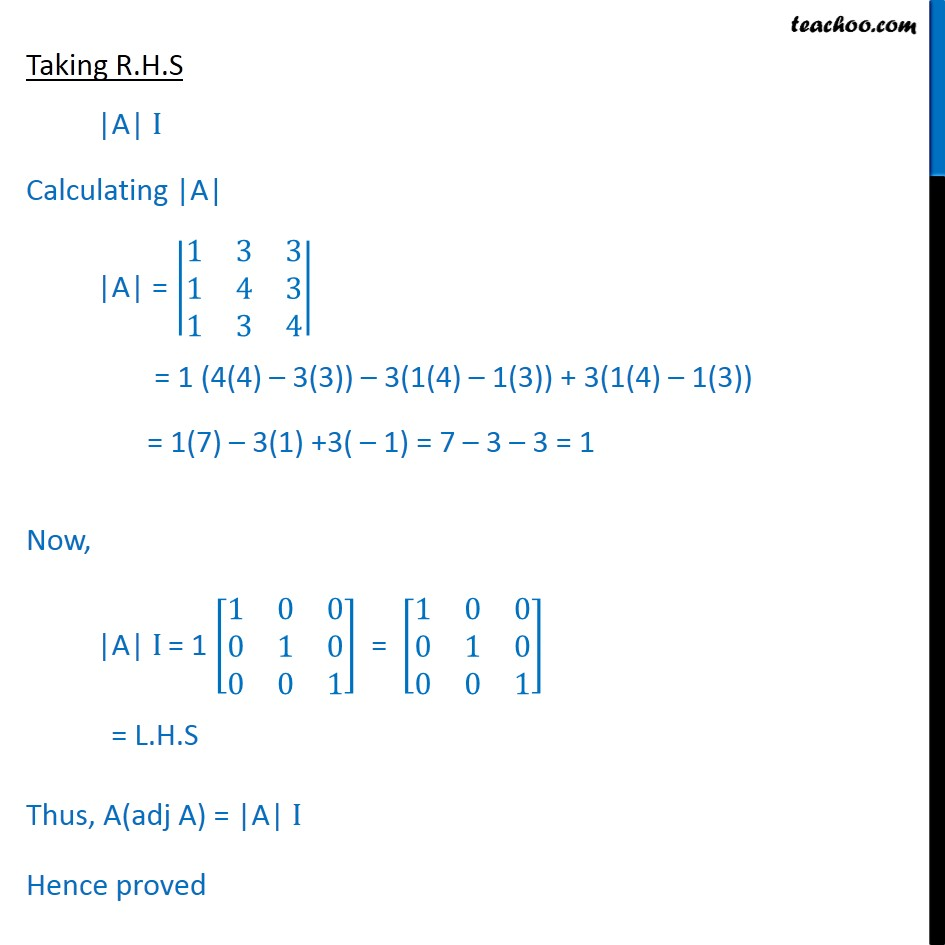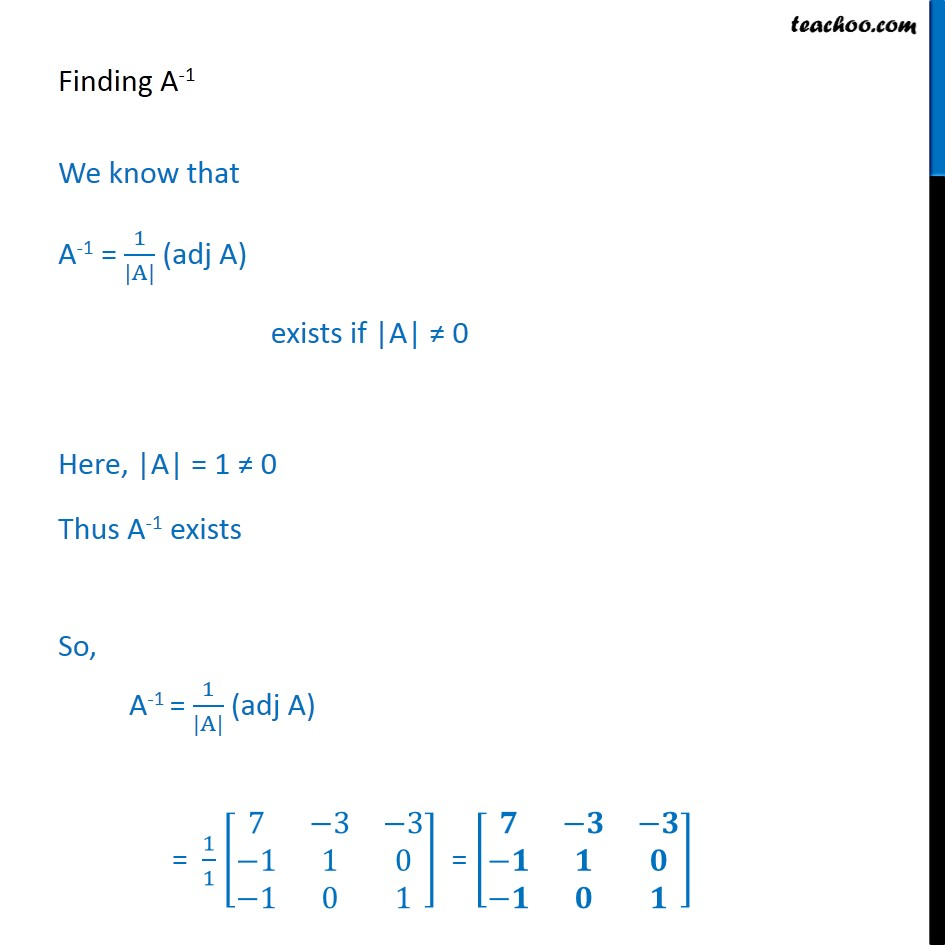1. Class 12
2. Important Question for exams Class 12
3. Chapter 4 Class 12 Determinants

Transcript

Example 24 If A = 1 3 3 1 4 3 1 3 4 , then verify that A adj A = |A| I. Also find A 1. Taking L.H.S A (adj A) First Calculating adj A adj A = A 11 A 21 A 31 A 12 A 22 A 32 A 13 A 23 A 33 Now, A = 1 3 3 1 4 3 1 3 4 M11 = 4 3 3 4 = 4(4) 3(3) = 7 M12 = 1 3 1 4 = 1(4) 1(3) = 1 M13 = 1 4 1 3 = 1(3) 1(4) = 1 M21 = 3 3 3 4 = 3(4) 3(3) = 3 M22 = 1 3 1 4 = 1(4) 1(3) = 1 Thus, adj (A) = A 11 A 21 A 31 A 12 A 22 A 32 A 13 A 23 A 33 = 7 3 3 1 1 0 1 0 1 Finding A (adj A) A adj (A) = 1 3 3 1 4 3 1 3 4 7 3 3 1 1 0 1 0 1 = 1 7 +3 1 +3( 1) 1 3 +3 1 +3(0) 1 3 +3 0 +3(1) 1 7 +4 1 +3( 1) 1 3 +4 1 +3(0) 1 3 +4 0 +3(1) 1 7 +3 1 +4( 1) 1 3 +3 1 +4(0) 1 3 +3 0 +4(1) = 7 3 3 3+3+0 3+0+3 7 4 3 3+4+0 3+0+3 7 3 4 3+3+0 3+0+4 = 1 0 0 0 1 0 0 0 1 Taking R.H.S |A| I Calculating |A| |A| = 1 3 3 1 4 3 1 3 4 = 1 (4(4) 3(3)) 3(1(4) 1(3)) + 3(1(4) 1(3)) = 1(7) 3(1) +3( 1) = 7 3 3 = 1 Now, |A| I = 1 1 0 0 0 1 0 0 0 1 = 1 0 0 0 1 0 0 0 1 = L.H.S Thus, A(adj A) = |A| I Hence proved Finding A-1 We know that A-1 = 1 |A| (adj A) exists if |A| 0 Here, |A| = 1 0 Thus A-1 exists So, A-1 = 1 |A| (adj A) = 1 1 7 3 3 1 1 0 1 0 1 =

Chapter 4 Class 12 Determinants

Class 12
Important Question for exams Class 12